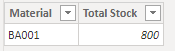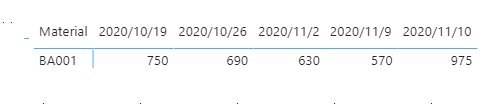cancel
Showing results for
Did you mean:Frequent Visitor

## Cumulative inventory Forecast

Good Morning Everyone!

I am new to power bi and i got stuck on inventory forecasting.

I am having problems forecasting the inventory value remaining by code on a weekly basis.

I have a table stating the On hand value and another table showing the forecast consuming the inventory every week.

I want to create a leading indicator graph which will allow me to start from the on hand inventory level and remove or add forecast each week.

The idea here is to then imput Safety stock levels or Upper and Lower Control Limits to each code so that we can quickly tell which products we will have problems on.

Here are pictures of what i would like as a final stage:ex 385 is the initial inventory, then next week the forecast is netting out 105 units so i will only have 280 on hand and so on and so forth.

Here are 2 pictures of the databases that i am trying to extrapolate this info from: Forecast, and On Hand.

ForecastOn HandIdeally i would love to run these 2 reports daily and see what products will go negative or below safety stock in a quickly manner.

**Products and quantities are made up for this exercise**

Thank you again for your help with this !

1 ACCEPTED SOLUTIONCommunity Support

I update my tables and my measure.

Forecast Table:On Hand Table:Safety Stock Table:Measures:

``````Stock =
VAR _CHANGE =
SUMX (
FILTER (
ALL ( Forecast ),
Forecast[Planned Dates] <= MAX ( Forecast[Planned Dates] )
&& Forecast[Material] = MAX ( Forecast[Material] )
&& Forecast[Storage Location] = MAX ( Forecast[Storage Location] )
),
Forecast[Quantity]
)
VAR _Total =
SUMX (
FILTER (
'On Hand',
'On Hand'[Material] = MAX ( Forecast[Material] )
&& 'On Hand'[Storage Location] = MAX ( 'Forecast'[Storage Location] )
),
'On Hand'[Total Stock]
)
RETURN
_CHANGE + _Total``````
``````Color =
IF (
[Stock]
>= CALCULATE (
MAX ( 'Safety stock'[SafeQT] ),
FILTER (
'Safety stock',
'Safety stock'[Material] = MAX ( Forecast[Material] )
&& 'Safety stock'[Storage Location] = MAX ( 'Forecast'[Storage Location] )
)
),
1,
0
)``````

Result:Here I have some question:

1. In your On Hand Table: Storage Location for BA000408001 is BA01. However in your Forecast Table, Storage Location is Blank when reqd quantity <0, and Storage Location is IT01 when reqd quantity>0. I couldn't find BA01 in your Forecast Table.

2. Does each material have the same planned dates like my sample?

Best Regards,

Rico Zhou

If this post helps, then please consider Accept it as the solution to help the other members find it more quickly.

5 REPLIES 5Frequent Visitor

@v-rzhou-msft  Thank you for your help !

I am now able to calculate what i needed.

The graphing part is very slow but i will try to fix this in other ways.

Thanks again!

AndreaCommunity Support

I think you want to accumulate forecast on Total stock by each date.

I build two sample table to have a test.

On Hand Table:Forecast Table:Measure:

``````Stock =
VAR _CHANGE = SUMX(FILTER(ALL(Forecast),Forecast[Planned Dates]<=MAX(Forecast[Planned Dates])&&Forecast[Material]=MAX(Forecast[Material])),Forecast[Quantity])
VAR _Total = SUMX(FILTER('On Hand','On Hand'[Material]=MAX(Forecast[Material])),'On Hand'[Total Stock])
Return
_CHANGE+_Total``````

Matrix:And you can flag the cell in other color if the stock less than safety stock by conditional formmatting.

I build a target table.Color measure:

``Color = IF([Stock]>= CALCULATE(MAX('Safety stock'[SafeQT]),FILTER('Safety stock','Safety stock'[Material]=MAX(Forecast[Material]))),1,0)``

Result:Best Regards,

Rico Zhou

If this post helps, then please consider Accept it as the solution to help the other members find it more quickly.Frequent Visitor

First of all i want to thank you for your help with this!

The dataset i sent you has different storage locations which i will need to be able to filter from in the measure you provided me with called " Stock" .

Also the dataset has multiple products so the 1 to many relationships that you provided me with for the connections between on Hand table and Forecast table won't work on this.

Let me know if you know how to fix this,

Best,

AndreaCommunity Support

I update my tables and my measure.

Forecast Table:On Hand Table:Safety Stock Table:Measures:

``````Stock =
VAR _CHANGE =
SUMX (
FILTER (
ALL ( Forecast ),
Forecast[Planned Dates] <= MAX ( Forecast[Planned Dates] )
&& Forecast[Material] = MAX ( Forecast[Material] )
&& Forecast[Storage Location] = MAX ( Forecast[Storage Location] )
),
Forecast[Quantity]
)
VAR _Total =
SUMX (
FILTER (
'On Hand',
'On Hand'[Material] = MAX ( Forecast[Material] )
&& 'On Hand'[Storage Location] = MAX ( 'Forecast'[Storage Location] )
),
'On Hand'[Total Stock]
)
RETURN
_CHANGE + _Total``````
``````Color =
IF (
[Stock]
>= CALCULATE (
MAX ( 'Safety stock'[SafeQT] ),
FILTER (
'Safety stock',
'Safety stock'[Material] = MAX ( Forecast[Material] )
&& 'Safety stock'[Storage Location] = MAX ( 'Forecast'[Storage Location] )
)
),
1,
0
)``````

Result:Here I have some question:

1. In your On Hand Table: Storage Location for BA000408001 is BA01. However in your Forecast Table, Storage Location is Blank when reqd quantity <0, and Storage Location is IT01 when reqd quantity>0. I couldn't find BA01 in your Forecast Table.

2. Does each material have the same planned dates like my sample?

Best Regards,

Rico Zhou

If this post helps, then please consider Accept it as the solution to help the other members find it more quickly.Helper I

@v-rzhou-msft I am having the same problem as the OP, however my planned dates are all different. They aren't the same for each type of material. How can I alter the "Stock" measure to account for this?